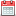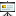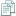# On localization of infinite spin particlesLQP 36 Foundations and Constructive Aspects of Quantum Field TheoryVincenzo Morinelli on May 29, 2015Morinelli.pdf

Given a unitary, positive energy, irreducible representation $U_0$ of the Poincar\'e group on a Hilbert space $\mathcal H$, one can associate a canonical real, closed, linear subspace $H(O)$ with any open region $O$ of the Minkowski spacetime $\mathbb R^{s+1}$. If $U_0$ is massless with infinite spin, Brunetti Guido and Longo showed that $H(O)$ is cyclic if $O$ is a spacelike cone (an unbounded region). It remained open whether $H(O)$ is non-trivial when $O$ is bounded. We show that indeed $H(O)= \{0\}$ for any bounded region $O$. This implies that the free field theory associated with infinite spin has no observables localized in bounded regions. In an interacting theory, if the vacuum vector is cyclic for a double cone local algebra, then the theory does not contain infinite-spin representations. These results hold under the natural assumption of the Bisognano-Wichmann property, and we present a counter-example (with continuous particle degeneracy) without this property where the conclusions fail. Our results hold true in any spacetime dimension $s+1$ where infinite spin representations exist, namely $s\geq 2$. (Based on a joint work with R.Longo and K.-H. Rehren)

Related papers:Where Infinite Spin Particles Are Localizable